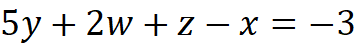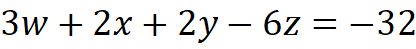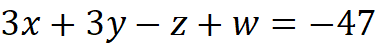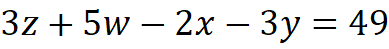1. Engineering
2. Computer Science
3. solve the following set of equations using numpys linear algebra...

# Question: solve the following set of equations using numpys linear algebra...

###### Question details

Solve the following set of equations using numpy’s linear algebra capabilities as well as on paper1. First, on paper, put the equation in the form Ax=b. Remember, each column of the coefficient matrix A represents coefficients of one of the variables. You’ll need to rewrite the equations above in that format before creating the A matrix.
2. Next, create arrays in Python for the A and b matrices.
3. Use numpy’s solve() function to solve for the x matrix. Record the results on paper.
4. Finally, verify the solution by printing the matrix product Ax to show that it is indeed b.
5. Just for fun, calculate A-1, the inverse of A. Show that the product A-1b gives the same result for x. Record the result on paper.
###### Solution by an expert tutor